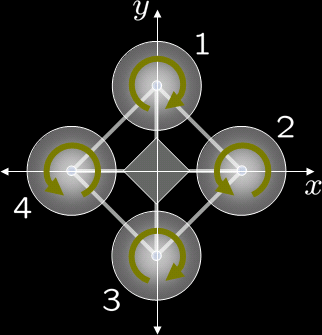## uavI'm making a UAV that is propelled by four rotors.

It uses a control loop running on a PIC composed of sensors, a Kalman filter, a PID controller, flight equations, and motors.

The PIC communicates via an RS-232 link with a Linux SBC.

#### Sensors

• 3 axis gyroscope
• 3 axis accelerometer
• 3 axis magnetometer
• barometer
• 4x infrared distance
• 5hz GPS

All the sensors are linked to the PIC.

#### Kalman filter

I found some very good examples online and on the SparkFun forums, but these have to be extended to many more states.

I've also implemented a simple one in a simulation to learn how to use it.

#### PID controller

The PID controllers recieve the current orientation from the Kalman Filter and the desired orientation from the SBC and combine them using the error, its integral, and its derivative to get the desired speed of each axis to get to the desired position.

There is a PID each for:

• Pitch
• Roll
• Yaw
• Altitude (output is rotated by roll and pitch)

#### Flight Equations

The UAV is modeled by these equations:

• Y = R1+R2+R3+R4
• T = R1-R2+R3-R4
• P = R1-R3
• R = R2-R4

Yaw, Thrust, Pitch, Roll - these are all the speed in that axis

R is the speed of each rotor. (rotor 2 and 4 are counter-rotating)

These equations can be solved to what I call the 'flight equations'.

• R1 = Y+T+P
• R2 = Y-T+R
• R3 = Y+T-P
• R4 = Y-T-R

### Motors

Four brushless motors will be used that are controlled by a custom I2C brushless controller.

### SBC

An NSLU2 is used as a SBC running Linux from a USB flash drive. The NSLU2 is modified to increase it's clock speed, make it always run when power is available, and add three extra USB ports to the normal two.

A flash stick and a USB Wifi adaptor are connected to the NSLU2.

The NSLU2 is also connected to several cameras that have door peepholes, which are used as wide-angle lenses. At most three can be used without adding an additional USB hub.

### Structure

There are three seperate boards:

• Motor/power - Four motor controllers, voltage regulators, and a battery charger
• IMU/flight controller - measures position and keeps aircraft stable, connected to Motor board via I2C link
• SBC - higher level actions, connected to IMU via serial link